# Computational Thinking Algorithms Programming Computer Science J 27602

• Slides: 21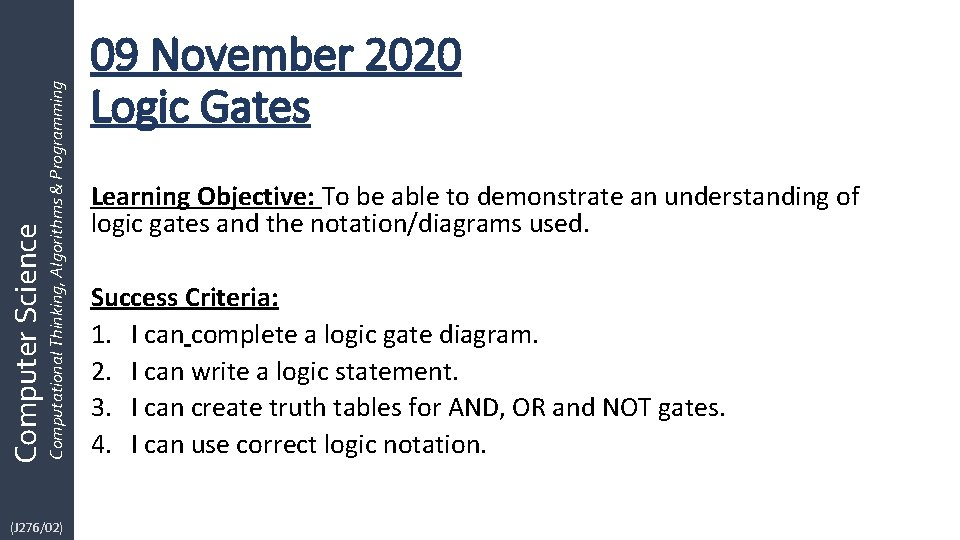Computational Thinking, Algorithms & Programming Computer Science (J 276/02) 09 November 2020 Logic Gates Learning Objective: To be able to demonstrate an understanding of logic gates and the notation/diagrams used. Success Criteria: 1. I can complete a logic gate diagram. 2. I can write a logic statement. 3. I can create truth tables for AND, OR and NOT gates. 4. I can use correct logic notation.Computational Thinking, Algorithms & Programming Computer Science (J 276/02) What is binary? • Binary is the representation of the ‘presence’ of electricity • If present or ‘on’ we use a: 1 • If absent or ‘off’ we use a: 0 • We can use this idea to change 1 and 0 states through the use of logic gates • Logic Gates take inputs and covert them to an output You can see why we need binary, logic and transistors in this video here: https: //www. youtube. com/watch? v=Gdqb. Lmd. Kgw 4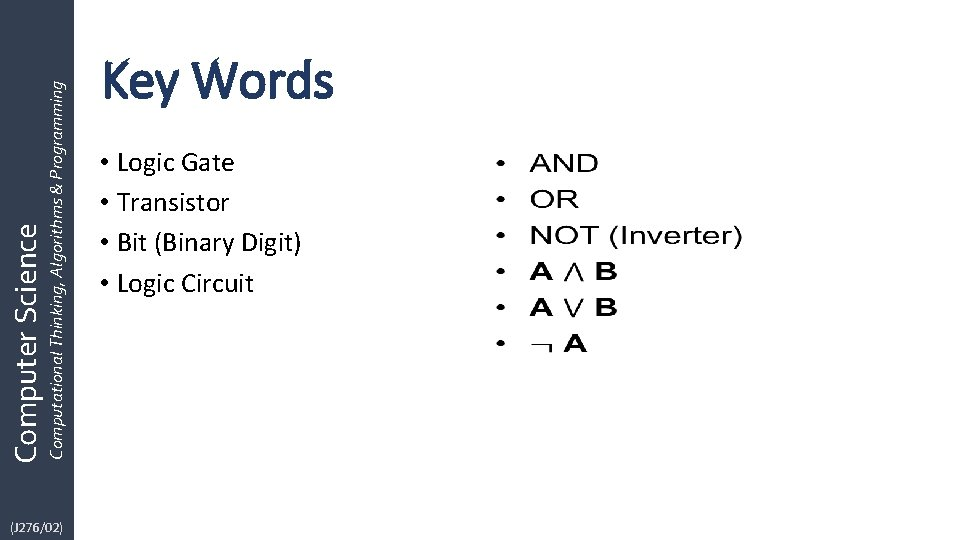Computational Thinking, Algorithms & Programming Computer Science (J 276/02) Key Words • Logic Gate • Transistor • Bit (Binary Digit) • Logic Circuit •Computational Thinking, Algorithms & Programming Computer Science (J 276/02) Logic Gates • Used to change bits and perform calculations within a computer • Created by using transistors • There are 3 basic logic gates: AND OR NOT • Every logic circuit can be made up from these 3 logic gatesComputational Thinking, Algorithms & Programming Computer Science (J 276/02) Truth Tables • • The output of Logic Gates can be shown through a Truth Table The Truth Table shows what an output will be, based on the inputs You will need to know the basic truth tables You will need to be able to construct a truth table from a logic statement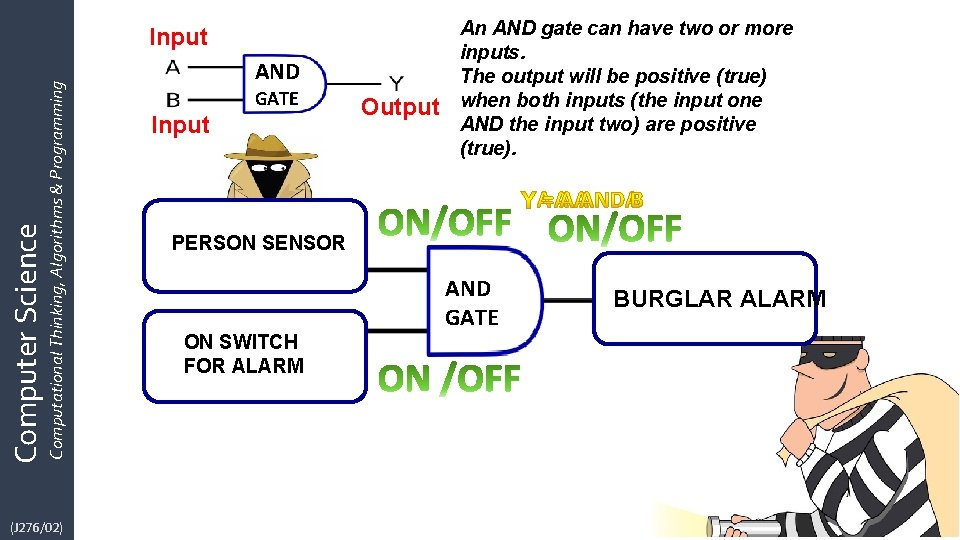Computer Science Computational Thinking, Algorithms & Programming Input (J 276/02) AND GATE Input Output An AND gate can have two or more inputs. The output will be positive (true) when both inputs (the input one AND the input two) are positive (true). PERSON SENSOR ON SWITCH c FOR ALARM AND GATE BURGLAR ALARM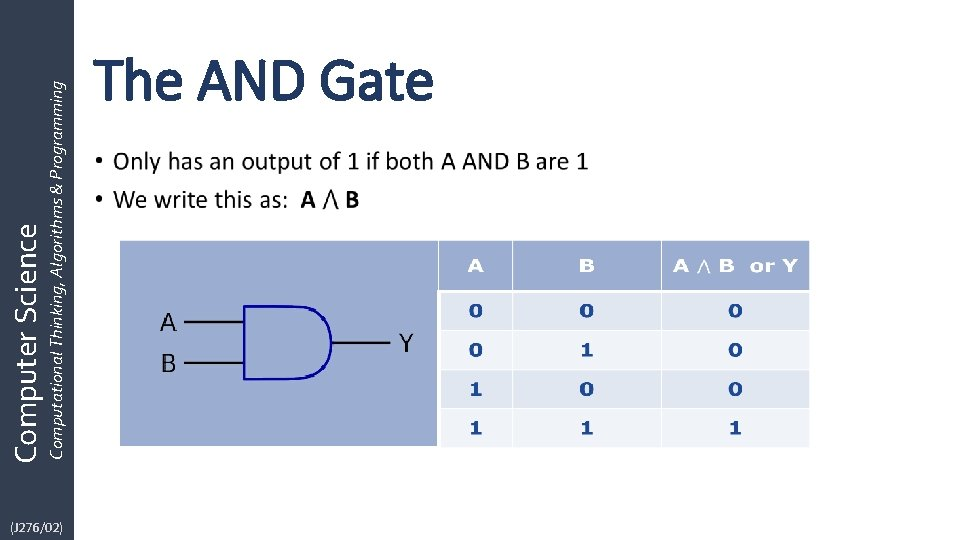(J 276/02) Computational Thinking, Algorithms & Programming Computer Science The AND Gate •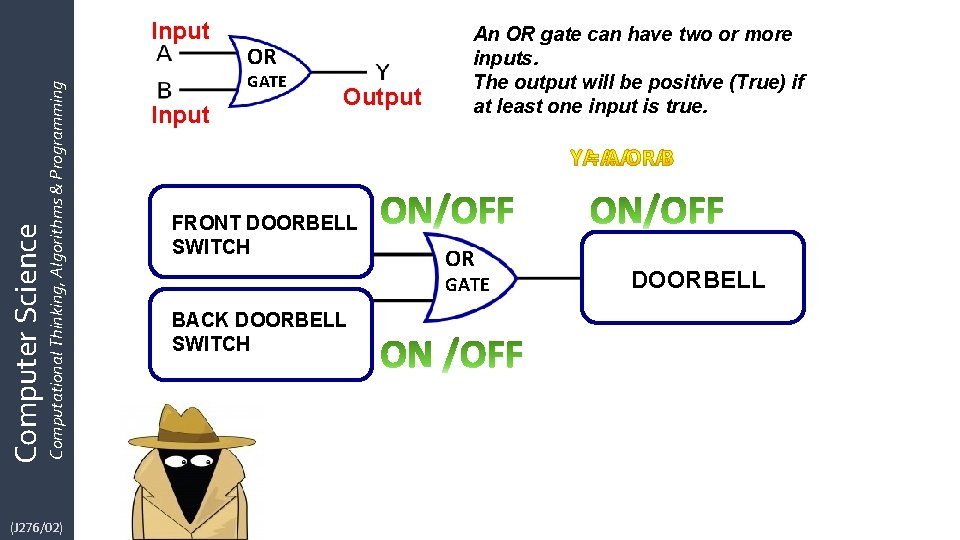Computer Science Computational Thinking, Algorithms & Programming Input (J 276/02) OR GATE Input Output FRONT DOORBELL SWITCH An OR gate can have two or more inputs. The output will be positive (True) if at least one input is true. OR GATE BACK DOORBELL SWITCH DOORBELL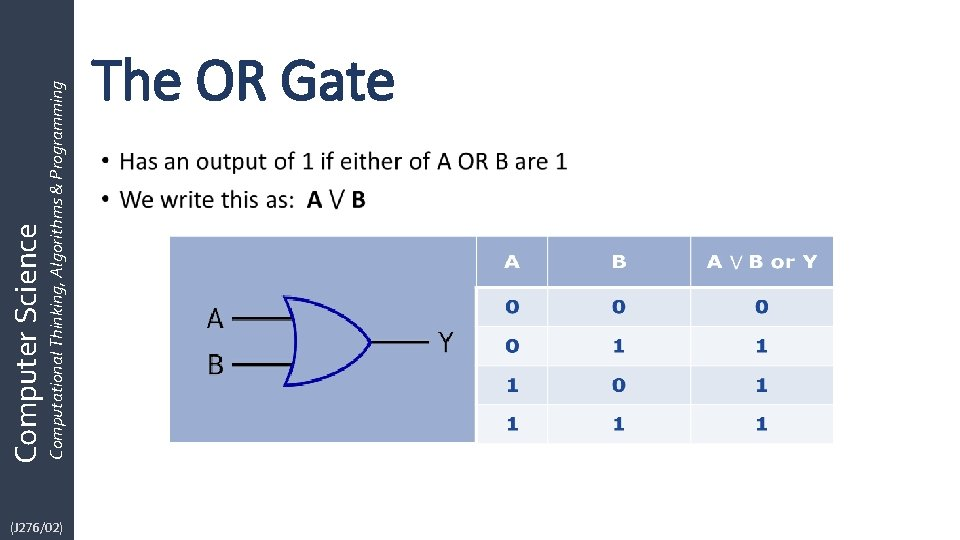(J 276/02) Computational Thinking, Algorithms & Programming Computer Science The OR Gate •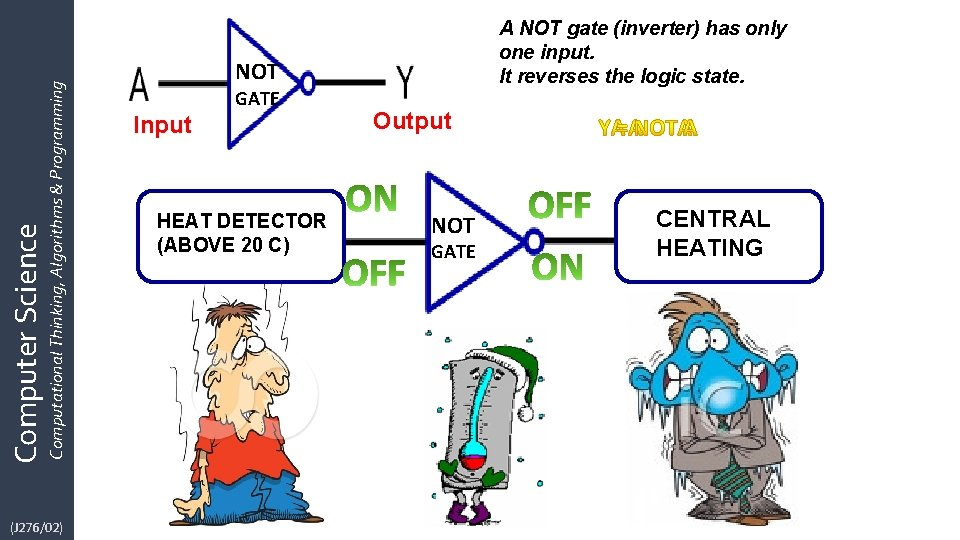Computational Thinking, Algorithms & Programming Computer Science (J 276/02) A NOT gate (inverter) has only one input. It reverses the logic state. NOT GATE Input HEAT DETECTOR (ABOVE 20 C) Output NOT GATE CENTRAL HEATINGComputational Thinking, Algorithms & Programming Computer Science (J 276/02) BEDROOM SENSOR ON or OFF ? OR GATE FRONT ROOM SENSOR AND GATE ON SWITCH FOR ALARM BURGLAR ALARM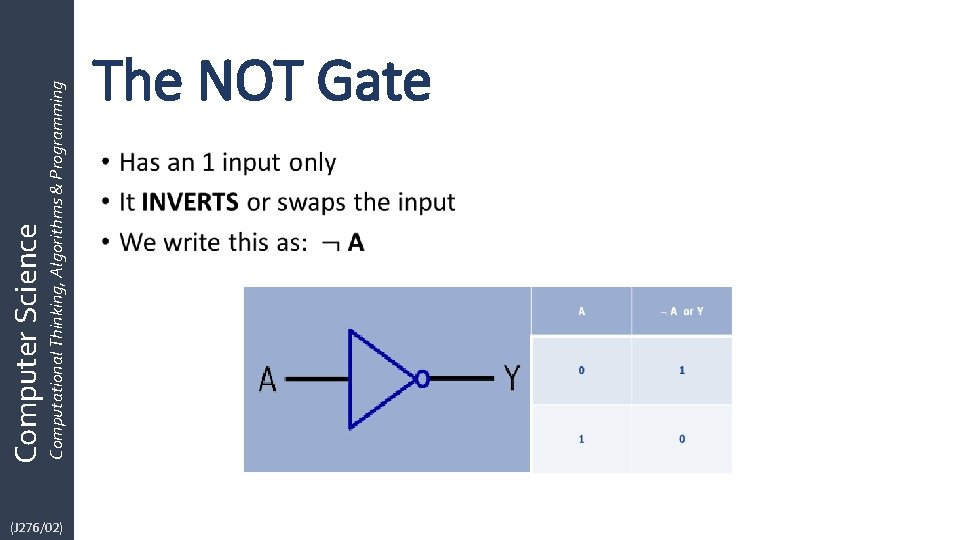(J 276/02) Computational Thinking, Algorithms & Programming Computer Science The NOT Gate •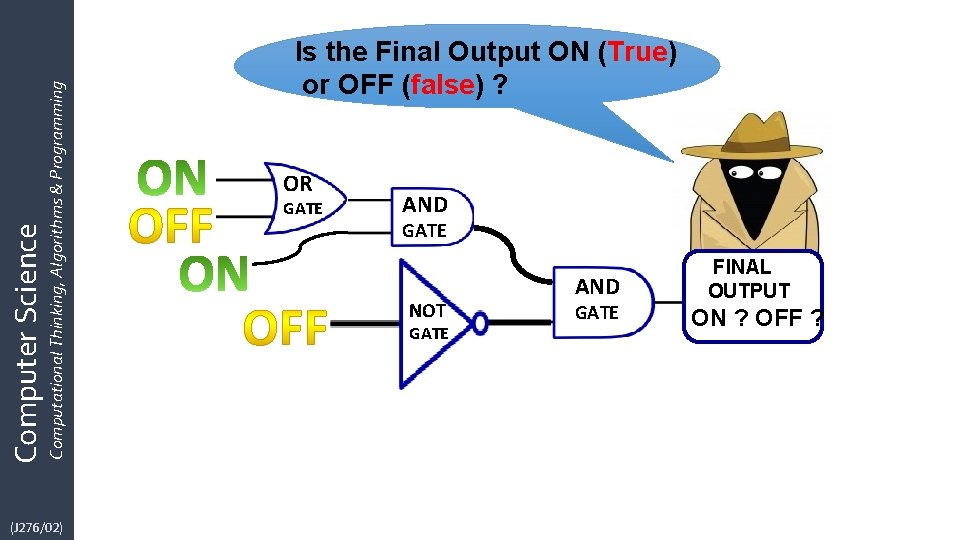Computational Thinking, Algorithms & Programming Computer Science (J 276/02) Is the Final Output ON (True) or OFF (false) ? OR GATE AND GATE NOT GATE AND GATE FINAL OUTPUT ON ? OFF ?Computational Thinking, Algorithms & Programming Computer Science (J 276/02) Activity 1: 1. ON OFF ON 4. OFF ? ON ? 5. 2. ON OFF ON ? ON 6. OFF ON OFF 3. ON OFF ? ? The output is ON or OFF ? ?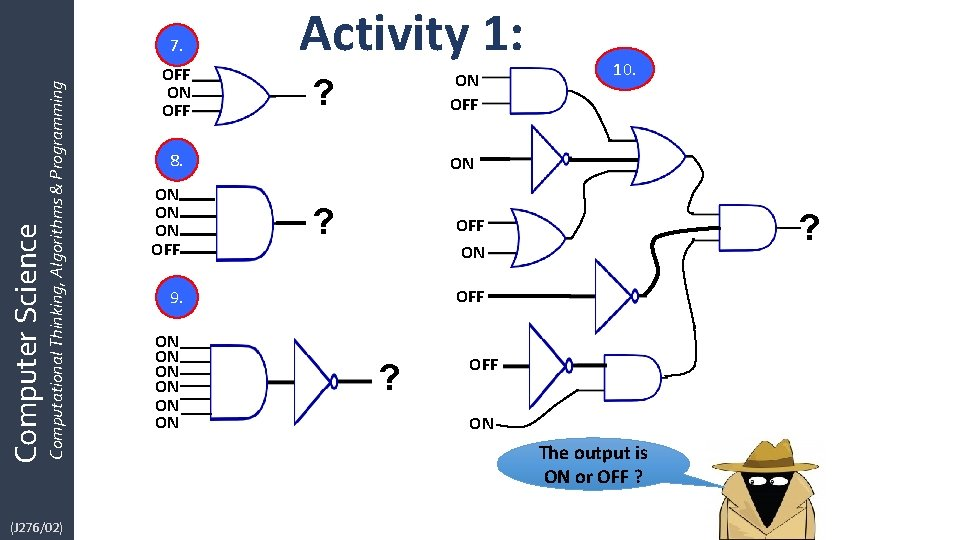Computer Science Computational Thinking, Algorithms & Programming 7. (J 276/02) OFF ON OFF Activity 1: ON OFF ? 8. ON ON ON OFF ON ? ? OFF ON OFF 9. ON ON ON 10. ? OFF ON The output is ON or OFF ?(J 276/02) Computational Thinking, Algorithms & Programming Computer Science Activity 3: Truth Tables • Complete the Table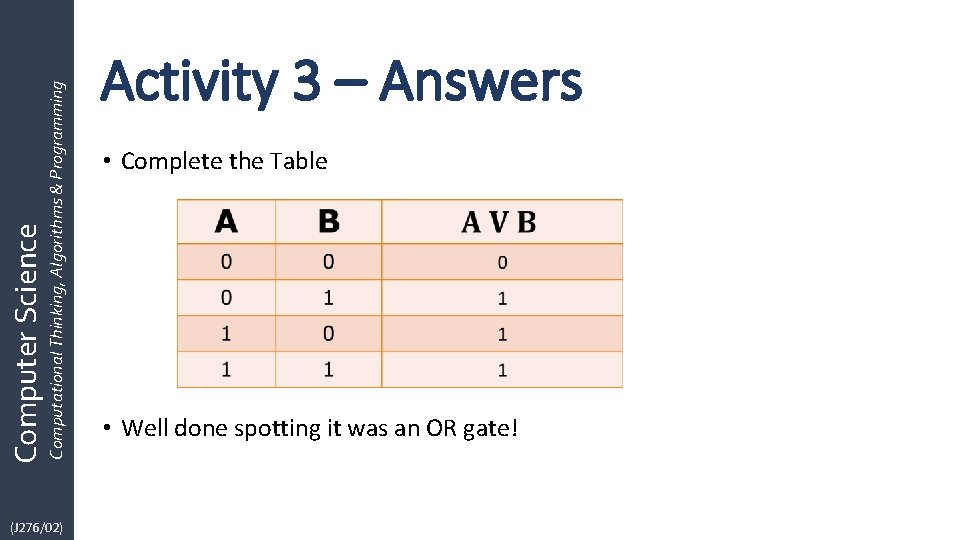Computational Thinking, Algorithms & Programming Computer Science (J 276/02) Activity 3 – Answers • Complete the Table • Well done spotting it was an OR gate!(J 276/02) Computational Thinking, Algorithms & Programming Computer Science Activity 4: Truth Tables •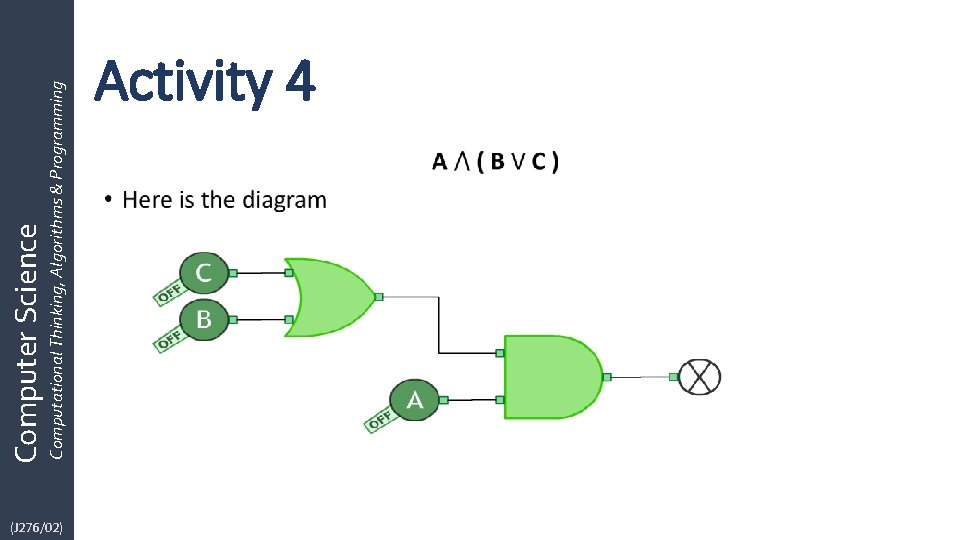(J 276/02) Computational Thinking, Algorithms & Programming Computer Science Activity 4 •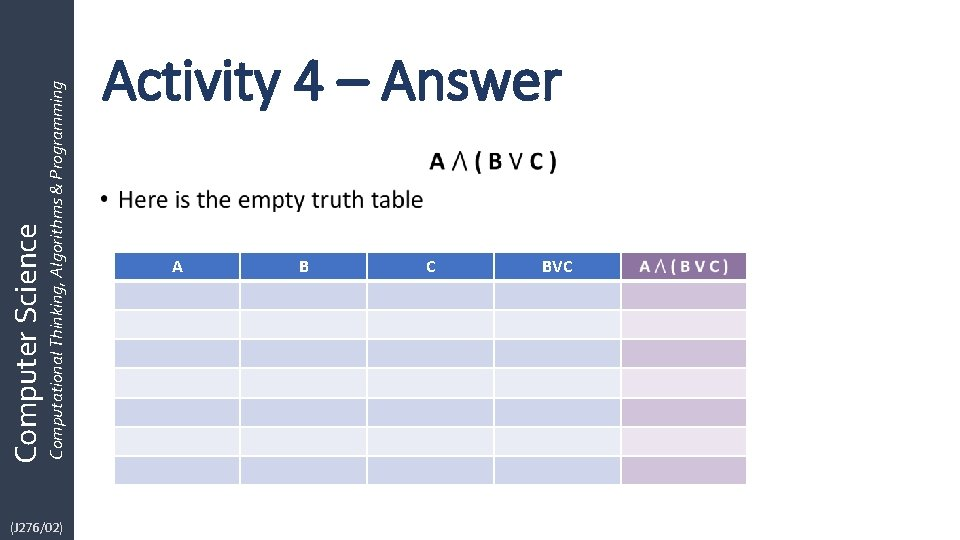(J 276/02) Computational Thinking, Algorithms & Programming Computer Science Activity 4 – Answer • A B C BVC(J 276/02) Computational Thinking, Algorithms & Programming Computer Science Activity 4 – Answer •